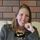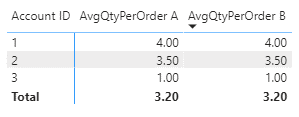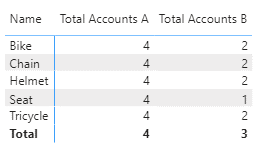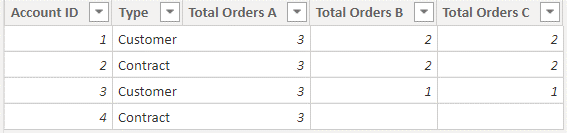cancel
Showing results for
Did you mean:## DAX Context Transition: Why it can be handy to use a [Measure] inside a Measure

Today I want to discuss an advanced reason for using a [Measure] inside another Measure. In beginner classes for DAX, I tell my students that they can use a [Measure] inside another Measure in order to:

• Refer to the same calculation across multiple Measures
Take the below data model as an example:Let's say we want to calculate the average quantity of items any given customer (Account) purchases in a single order, based on all their past orders. In order to get AvgQtyPerOrder for a given Account, I simply need to divide their total quantity by their total orders. To calculate this using DAX, I have a few options.

### Option A: All calculations in one measure

AvgQtyPerOrder A =
DIVIDE (
SUM ( SalesOrderDetails[Qty] ),
DISTINCTCOUNT ( SalesOrderDetails[Order Number] )
)

### Option B: Break calculations into three smaller measures

Total Qty =
SUM ( SalesOrderDetails[Qty] )

Total Orders =
DISTINCTCOUNT ( SalesOrderDetails[Order Number] )

AvgQtyPerOrder B =
DIVIDE ( [Total Qty], [Total Orders] )

As you can see in the image below, both options return the same result:Option A is great because it's transparent and I can easily see exactly what calculations are happening within the Measure, but it's not exactly easy to read, and it's not how my brain works when authoring formulas. I personally find it easier to break the DAX into bite size chunks.

Option B gives us those bite sized chunks and when all formulas for each measure are viewed side by side like this, it's much easier to digest.

It's also possible to go on to use [Total Qty] or [Total Orders] in other measures, without needing to rewrite the formula for each measure. For example, we might use [Total Orders]  to calculate the total number of customers (Accounts) who have purchased something from us:

Total Accounts B =
COUNTROWS ( FILTER ( Account, [Total Orders] > 0 ) )

The danger with using Option B is that we lose that transparency and [Total Orders] is now masked in a Measure name. Without checking the formula for [Total Orders] we can't be 100% certain what [Total Accounts B] is actually doing.

Okay, so we can do Option A instead, right?

Total Accounts A =
COUNTROWS (
FILTER ( Account, DISTINCTCOUNT ( SalesOrderDetails[Order Number] ) > 0 )
)

All we've done is replace [Total Orders] with its definition DISTINCTCOUNT ( SalesOrderDetails[Order Number] ) so we should get the same result, right?

WRONG! Here's the result we get when we place are two measures in a matrix with Product Name:We can see that Option B is giving us the correct result of only the accounts who bought that product, and the Total gives the number of Accounts who have bought anything. Option A simply gives the total number of Accounts in the Accounts table for all rows in the matrix.

# WHY does it matter if we use a measure inside a measure, or simply define the measure instead?

The answer is due to the Context Transition that happens when we use DAX filter functions.

• FILTER
• ALL
• ALLEXCEPT
• CALCULATE
• etc.

## DAX Context

DAX has two contexts that we must ALWAYS take into consideration when authoring DAX formulas:
• Row Context
• Filter Context
When authoring MEASURES, we are always working within the current Filter Context provided by the data model, the visuals, slicers, and report level filters. When authoring COLUMNS, we work with the Row Context provided by the table. And of course, there's lots of cross-over too, like when:
• using Iterators (such as SUMX) within a MEASURE to change the Filter Context to a Row  Context of the Table defined in the DAX expression
• using CALCULATE within a COLUMN to change the Row Context of the data model table to a Filter Context that would be present in a MEASURE used within a report matrix or table visual containing the same data
• using table Filter Functions (such as FILTER) within a MEASURE to change the Filter Context to a Row Context of the Table defined in the DAX expression
We call that cross-over Context Transition

## Context Transition with filtering functions

Recall, we're still trying to figure out why the following MEASURES give 2 different results when used in a matrix with Product[Name].Total Accounts A =
COUNTROWS (
FILTER ( Account, DISTINCTCOUNT ( SalesOrderDetails[Order Number] ) > 0 )
)

Total Accounts B =
COUNTROWS ( FILTER ( Account, [Total Orders] > 0 ) )

Note, these measures are being used inside a FILTER() function. The FILTER function requires two arguments:
FILTER(<Table>, <Filter>)

The Table as its first argument applies a Row Context to whatever expression is in the <Filter> argument. We know that Row Context applies in calculated COLUMNS.

To see what's going on in our two MEASURES, we're going to take a quick look at some calculated COLUMNS in order to simulate the Context Transition applied by the FILTER function.

Both Option A and B are applying a FILTER on the Account table, so let's try jumping into the Account table and create a couple of calculated COLUMNS to see the difference. The difference between Option A and Option B was how to calculate Total Orders:

Total Orders A = DISTINCTCOUNT ( SalesOrderDetails[Order Number] )

Total Orders B = [Total Orders]

Here's the result in the calculated COLUMNS in Account table:Option A using DISTINCTCOUNT provides the same result for every row in the table. This should come as no surprise, we know not to use aggregate functions (such as COUNT) within a calculated column, as it doesn't provide the correct context.

Option B using the [Total Orders] measure interestingly provides a different result for each row in the table. It does this because the MEASURE provides a Filter Context and our data model relationships allow this transition to happen.

The same thing happens within our original MEASURES; when we use the [Total Orders] measure, we get the context transition we're looking for. When we use DISTINCTCOUNT, we get incorrect Row Context.

## Mystery solved!

So, in summary, we can now update our beginner rationale for [Measure] reference inside Measures with the more complex rationale:

Use a [Measure] inside another Measure in order to:
• Refer to the same calculation across multiple Measures
• Apply a Context Transition when using filtering functions

## BONUS: Context Transition with CALCULATE

Just for fun, we can take this one step further and add an option C into the mix. Let's start with a review of CALCULATE and what it does. From Microsoft Docs regarding CALCULATE:

Definition: Evaluates an expression in a context that is modified by the specified filters.
Remarks: If the data has been filtered, the CALCULATE function changes the context in which the data is filtered and evaluates the expression in the new context that you specify. For each column used in a filter argument, any existing filters on that column are removed, and the filter used in the filter argument is applied instead.
Okay, so we can use CALCULATE instead of a MEASURE to achieve the desired result with greater transparency of our calculation.

Note that the below calculated COLUMN in the Account table gives the correct numbers, just as using the [Total Orders] measure.

Total Orders C =
CALCULATE ( DISTINCTCOUNT ( SalesOrderDetails[Order Number] ) )So, if we substitute the expression for Total Orders C into our original Measure, we should get the correct numbers:

Total Accounts C =
COUNTROWS (
FILTER (
Account,
CALCULATE ( DISTINCTCOUNT ( SalesOrderDetails[Order Number] ) ) > 0
)
)And we do get the correct numbers! CALCULATE enables us to change the context of the Total Accounts measure and achieve the complex results we're looking for.